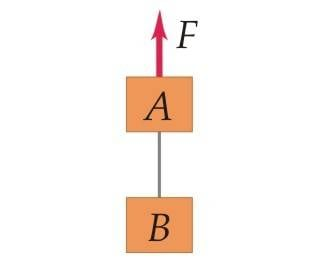# Force Body Diagram Physics problem: Finding mass of boxes

## Homework Statement

Two boxes, A and B, are connected to each end of a light vertical rope, as shown in the following figure. A constant upward force 74.0 N applied to box A. Starting from rest, box B descends 10.6 m in 3.00 s. The tension in the rope connecting the two boxes is 33.0 NThis is the given figure.

The given information is: F=74.0N
Distance traveled for box B=10.6m
Time:3.00s
Tension=33.0N

F=ma

## The Attempt at a Solution

Wrote out the formulas for each of the parts, since the problem is asking to solve for box B first, I attempted to solve for that. The forces that I assumed were acting on box B are T, F of gravity, and possibly(?) the force of box A, since they are connected by the string. I suppose where I am confused most at this point is how the diagram looks. Since the problem is saying that box B descends, is it moving in the opposite direction of A?

Buzz Bloom
Gold Member
Hi Stendal:

The problem statement in Section1 of your pose doesn't specify what the problem is you are trying to solve. Can you add this piece of information?

Regards,
Buzz

Hi Stendal:

The problem statement in Section1 of your pose doesn't specify what the problem is you are trying to solve. Can you add this piece of information?

Regards,
Buzz

Sorry about that, I am supposed to be solving for the masses of both of the boxes of A and B. However, I actually just figured out how to do it. I'll post how to do it just to show the method of thought used to figure it out for future use.

I assumed that the acceleration for A and B were both constant and equal to each other, so I found the acceleration by using a set of 1 dimensional equations. First finding the velocity, and solving for the acceleration, which turned out to be 2.4m/s^2.

With the summation of the forces, I set the force of gravity and tension equal the the mass of B multiplied by the acceleration. Since in that, the only unknown variable is the mass, you can manipulate the equation to figure out the mass, which for me was about 4.43 kg.

Given the assumptions of acceleration above, you can set up the summation of forces for A similar to B, with the exception of having the constant force being applied to A. Manipulation the equation again, the mass came out to be around 5.51kg.